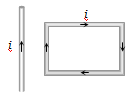An $\mathrm{\alpha }-$ particle travels in a circular path of radius 0.45 m in a magnetic field  with a speed of  . The period of revolution of the $\mathrm{\alpha }-$ particle is :

(a)   sec          (b)  $1.1×{10}^{-6}$ sec

(c)  $1.1×{10}^{-7}$ sec           (d)  $1.1×{10}^{-8}$ sec

Concept Questions :-

Lorentz force
High Yielding Test Series + Question Bank - NEET 2020

Difficulty Level:

A deutron of kinetic energy 50 keV is describing a circular orbit of radius 0.5 metre in a plane perpendicular to magnetic field $\stackrel{\to }{\mathrm{B}}$. The kinetic energy of the proton that describes a circular orbit of radius 0.5 metre in the same plane with the same $\stackrel{\to }{\mathrm{B}}$ is :

(a) 25 kev                           (b) 50 kev

(c) 200 kev                          (d) 100 kev

Concept Questions :-

Lorentz force
High Yielding Test Series + Question Bank - NEET 2020

Difficulty Level:

A rectangular loop carrying a current i is situated near a long straight wire such that the wire is parallel to the one of the sides of the loop and is in the plane of the loop. If a steady current I is established in wire as shown in figure, the loop will(a) Rotate about an axis parallel to the wire

(b) Move away from the wire or towards right

(c) Move towards the wire

(d) Remain stationary

Concept Questions :-

Current carrying loop : Force and torque
High Yielding Test Series + Question Bank - NEET 2020

Difficulty Level:

Two thin long parallel wires separated by a distance b are carrying a current i amp each. The magnitude of the force per unit length exerted by one wire on the other is

(a) $\frac{{\mu }_{0}{i}^{2}}{{b}^{2}}$                                  (b) $\frac{{\mu }_{0}{i}^{2}}{2\mathrm{\pi b}}$

(c) $\frac{{\mu }_{0}i}{2\mathrm{\pi b}}$                                   (d) $\frac{{\mu }_{0}i}{2{\mathrm{\pi b}}^{2}}$

Concept Questions :-

Force between current carrying wires
High Yielding Test Series + Question Bank - NEET 2020

Difficulty Level:

To make the field radial in a moving coil galvanometer :

(a) The number of turns in the coil is increased

(b) Magnet is taken in the form of horse-shoe

(c) Poles are cylindrically cut

(d) The coil is wounded on the aluminum frame

Concept Questions :-

Moving coil galvanometer

Difficulty Level:

In a moving coil galvanometer, the deflection of the coil $\theta$ is related to the electrical current i by the relation

(a) $i\propto \mathrm{tan}\theta$                  (b) $i\propto \theta$

(c) $i\propto {\theta }^{2}$                     (d) $i\propto \sqrt{\theta }$

Concept Questions :-

Moving coil galvanometer
High Yielding Test Series + Question Bank - NEET 2020

Difficulty Level:

A moving coil galvanometer has N number of turns in a coil of effective area A, it carries a current I. The magnetic field B is radial. The torque acting on the coil is

(a) $N{A}^{2}{B}^{2}I$                           (b) $NAB{I}^{2}$

(c) ${N}^{2}ABI$                             (d) $NABI$

Concept Questions :-

Moving coil galvanometer
High Yielding Test Series + Question Bank - NEET 2020

Difficulty Level:

If a particle of charge ${10}^{-12}$ coulomb moving along the  direction with a velocity ${10}^{5}$ m/s experiences a force of ${10}_{}^{-10}$ newton in $\stackrel{^}{y}$- direction due to magnetic field, then the minimum magnetic field is

(a)  $6.25×{10}^{3}$ tesla in $\stackrel{^}{z}$- direction

(b)  ${10}^{-15}$  tesla in $\stackrel{^}{z}$- direction

(c)  $6.25×{10}^{-3}$ tesla in $\stackrel{^}{z}$- direction

(d)  ${10}^{-3}$ tesla in $\stackrel{^}{z}$- direction

Concept Questions :-

Lorentz force
High Yielding Test Series + Question Bank - NEET 2020

Difficulty Level:

A small coil of N turns has an effective area A and carries a current I. It is suspended in a horizontal magnetic field $\stackrel{.}{B}$ such that its plane is perpendicular to $\stackrel{.}{B}$. The work done in rotating it by $180°$ about the vertical axis is

(a) $NAIB$                               (b) $2NAIB$

(c) $2\mathrm{\pi NAIB}$                          (d) $4\mathrm{\pi }NAIB$

Concept Questions :-

Magnetic moment
High Yielding Test Series + Question Bank - NEET 2020

Difficulty Level:

An electron moves with a constant speed v along a circle of radius r. Its magnetic moment will be (e is the electron's charge)

(a) evr                        (b) $\frac{1}{2}$evr

(c) ${\mathrm{\pi r}}^{2}$ev                     (d) 2$\mathrm{\pi }$rev

Concept Questions :-

Magnetic moment